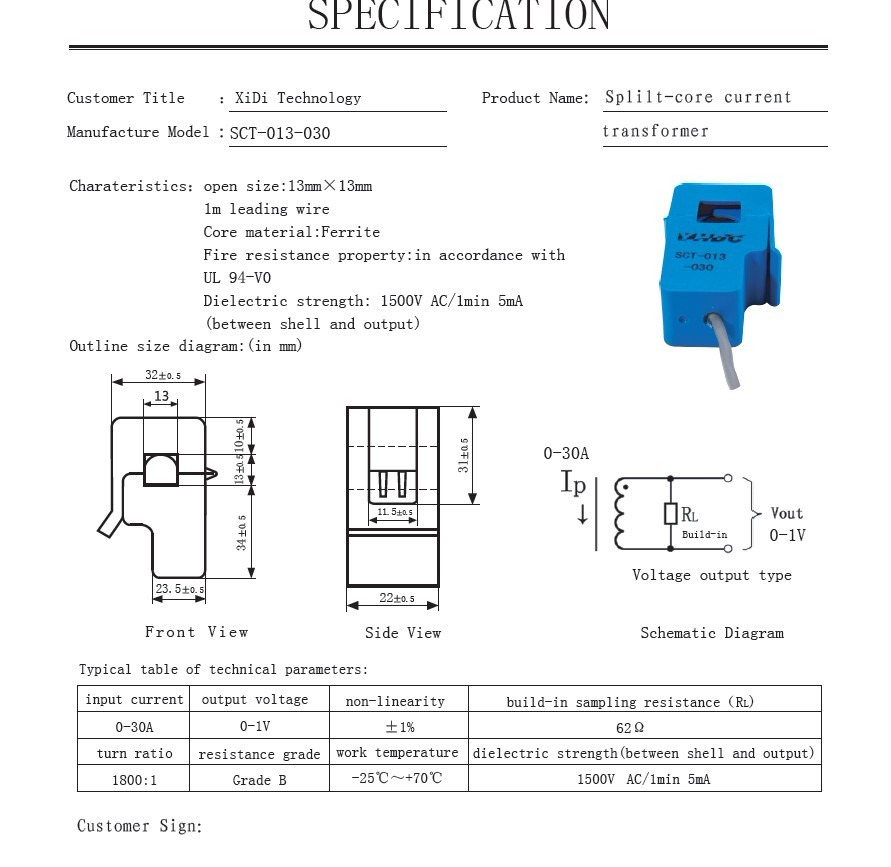# CURRENT SENSOR NOT WORKING.

The current sensor on my Spider is mot working.
I used a timer to get the value every 200 miliseconds.

```double current = currentSensor.GetCurrentReading(); string s = "current:" + current.ToString(); Debug.Print(s);```

Just connected the current sensor to one of the wires of my lamp and got the current values below. It is not very useful since the values are all over. It didn’t matter wether I hooked it to line or neutral.

current:0.0966796875
current:0.38671875
current:0.4833984375
current:0
current:0.0966796875
current:0.29003906249999994
current:0.0966796875
current:0.4833984375
current:0.58007812499999989
current:0.29003906249999994
current:0.38671875
current:0.58007812499999989
current:0.29003906249999994
current:0.29003906249999994
current:0

Anyone with luck?

Hi there,

What type current sensor do you work with?
Because the current sensor module is using in detect the big current(above 100ma)
So that might be why you can get a correct value.

Yuri

I’m using the seeed studio current sensor module for Fez Spider.
I attached it to one wire(line or neutral) on my 60Watt lamp and the current shows as zero most of the time. Once in a while it would jump up and then back to zero. Not very useful.

Hi there,

The lamp current is too low, according your 60 watt UI=W, so the current should be around 300 mA.That is too low.

And current also read the instantaneous value. Then via driver to out put value on PC.
So this module is for 100A current.
seeedstudio.com/depot/curren … ?cPath=203

Actually, more like 500mA since we have 120V in the US.
Here’s a link to the module I have.
http://www.ghielectronics.com/catalog/product/324What is the use of a current sensor that works for 100A AC and not work with low currents normally found in a home. If you had specified all the specifications correctly, I would not have ordered it. What a scam.

Hi there,

Sorry for my mistake.

Your Voltage is 120v and the current sensor with Gadgeteer is MAX for 30A.

But it is still have some problem with low current.
Here first to give you the sensor datasheet.

Hi, everyone , maybe the seeed gays do not express this module and it’s driver clearly.

1. The current sensor module is an current transformer that has a turn ratio of 1800:1 , the current measured can be upto 30A. it also has a build-in sampling resistance of 62Ω, that is, when the input current is 30A, the output voltage would be : 30/180062≈1V. as the spider ADC ref is 3.3v and the ADC is 10bit , so the minimum resolution would be :303.3/1024≈100mA. Attached the datasheet of the module.
2. Actully , this module is just a transformer, it can not convert AC to DC or measure the AC current directly. If in use of measuring AC, we need to sample the voltage repeatly, to find out the max of the sample voltage, the numbers of the ADC should be enough to guarantee the peak value or value close to the peak was sampled (that is to say, the sample times should be more than a period of the signal). like the code below:

```public double GetCurrentReading() { double current = 0; double max = 0; for (int i = 0; i < 200; i++) { current = analogInput.ReadVoltage(); if (max < current) { max = current; } } return max * 30; } ```
we sampled 200 times and finds out the max, and then multiply with the ratio, to get the peak of the AC current. If we need the equivalencevalue, you can divide it with a 1.414…

in this way, I get the current of my air conditioner
10.1513671875
10.0546875
10.0546875
10.1513671875
3. as the spider kit in my hand, if I sample the voltage in my user application, the ADC speed is too low, but if I do the sample in the driver, it is much more faster. So , I think , how about modify the module driver like this: set 2 functions , one is GetDCCurrent() and the other is GetACCurrent(), in the GetDCCurrent(), only one ADC was needed. In the GetACCurrent(), hundreds of ADC was needed to find out the peak value. In this way, the driver would be more easy to use.
4. maybe we seeedstudio needs sometime to re-design the driver, before the new version release, maybe you can try to modify the driver by yourself, just modify the function: getcurrentreading() like above, to sample the signal repeatly and finds out the max , as i measured , 200 times would be ok. by this way , you can measure and monitor AC current with a 100mA resolution.
thanks.